### Torque-Slip Characteristics

As the induction motor is located from no load to full load, its speed decreases hence slip increases. Due to the increased. load, motor has to produce more torque to satisfy load demand. The torque ultimately depends on slip as explained earlier. The behaviour of motor can be easily judged by sketching a curve obtained by plotting torque produced against slip of induction motor. The curve obtained by plotting torque against slip from s = 1 (at start) to s = 0 (at synchronous speed) is called torque-slip characteristics of the induction motor. It is very interesting to study the nature of torque-slip characteristics.
We have seen that for a constant supply voltage,  E2 is also constant. So we can write torque equations as,

Now to judge the nature of torque-slip characteristics let us divide the slip range (s = 0 to s = 1) into two parts and analyse them independently.

i) Low slip region :
In low slip region, 's' is very very small. Due to this, the term (s X2)2 is so small as compared to R22 that it can be neglected.

Hence in low slip region torque is directly proportional to slip. So as load increases, speed decreases, increasing the slip. This increases the torque which satisfies the load demand.
Hence the graph is straight line in nature.
At N = Ns , s = 0 hence T = 0. As no torque is generated at N = Ns, motor stops if it tries to achieve the synchronous speed. Torque increases linearly in this region, of low slip values.
ii) High slip region :
In this region, slip is high i.e. slip value is approaching to 1. Here it can be assumed that the term R22 is very very small as compared to (s X2)2. Hence neglecting from the denominator, we get

So in high slip region torque is inversely proportional to the slip. Hence its nature is like rectangular hyperbola.
Now when load increases, load demand increases but speed decreases. As speed decreases, slip increases. In high slip region as T α1/s, torque decreases as slip increases.
But torque must increases to satisfy the load demand. As torque decreases, due to extra loading effect, speed further decreases and slip further increases. Again torque decreases as T  α1/s hence same load acts as an extra load due to reduction in torque produced. Hence speed further drops. Eventually motor comes to standstill condition. The motor can not continue to rotate at any point in this high slip region. Hence this region is called unstable region of operation.
So torque - slip characteristics has two parts,
1. Straight line called stable region of operation
2. Rectangular hyperbola called unstable region of operation.
Now the obvious question is upto which value of slip, torque - slip characteristics represents stable operation ?
In low slip region, as load increases, slip increases and torque also increases linearly. Every motor has its own limit to produce a torque. The maximum torque, the  motor can produces as load increases is Tm which occurs at s = sm. So linear behaviour continues till s = sm.
If load is increased beyond this limit, motor slip acts dominantly pushing motor into high slip region. Due to unstable conditions, motor comes to standstill condition at such a load. Hence i.e. maximum torque which motor can produce is also called breakdown torque or pull out torque. So range s = 0 to s = sm is called low slip region, known as stable region of operation. Motor always operates at a point in this region. And range s = sm to s = 1 is called high slip region which is rectangular hyperbola, called unstable region of operation. Motor can not continue to rotate at any point in this region.
At s = 1, N = 0 i.e. start, motor produces a torque called starting torque denoted as Tst.
The entire torque - slip characteristics is shown in the Fig. 1.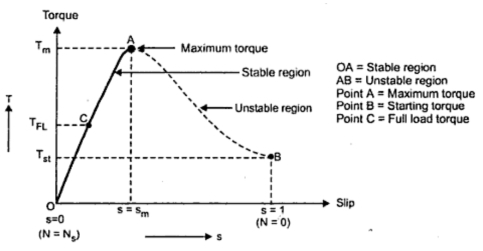Fig. 1  Torque speed characteristics

1.1 Full load torque
When the load on the motor increases, the torque produced increases as speed decreases and slip increases. The increases torque demand is satisfied by drawing motor current from the supply.
The load which motor can drive safely while operating continuously and due to such load, the current drawn is also within safe limits is called full load condition of motor. When current increases, due to heat produced the temperature rise. The safe limit of current is that which when drawn for continuous operation of motor, produces a temperature rise well within the limits. Such a full load point is shown on the torque-slip characteristics torque as TF.L.
The interesting thing is that the load on the motor can be increased beyond point C  till maximum torque condition. But due to high current and hence high temperature rise there is possibility of damage of winding insulation, if motor is operated for longer time duration in this region i.e. from point C  to B. But motor can be used to drive loads more than full load, producing torque upto maximum torque for short duration of time. Generally full load torque is less than the maximum torque.
So region OC  upto full load condition allow motor operation continuously and safely from the temperature point pf view. While region CB  is possible to achieve in practice but only for short duration of time and not for continuous operation of motor. This is the difference between full load torque and the maximum or breakdown torque. The breakdown torque is also called stalling torque.
1.2 Generating and Braking Region
When the slip lies in the region 0 and 1 i.e. when 0 ≤s ≤1, the machine runs as a motor which is the normal operation. The rotation of rotor is in the direction of rotating field which is developed by stator currents. In this region it takes electrical power from supply lines and supplies mechanical power output. The rotor speed and corresponding torque are in same direction.
When the slip is greater than 1, the machine works in the braking mode. The motor is rotated in opposite direction to that of rotating field. In practice two of the stator terminals are interchanged which changes the phase sequence which in turn reverses the direction of rotation of magnetic field. The motor comes to quick stop under the influence of counter torque which produces braking action. This method by which the motor comes to rest is known as plugging. Only care is taken that the stator must be disconnected from the supply to avoid the rotor to rotate in other direction
To run the induction machine as a generator, its slip must be less than zero i.e. negative. The negative slip indicates that the rotor is running at a speed above the synchronous speed. When running as a generator it takes mechanical energy and supplies electrical energy from the stator.
Thus the negative slip, generation action takes place and nature of torque - slip characteristics reverses in this generating region.
The Fig.2 shows the complete torque - slip characteristics showing motoring, generating and the braking region.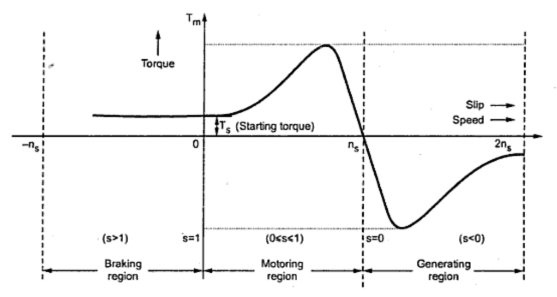Fig. 2  Regions of torque - slip characteristics

1.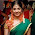sir your explanation is very nice but could you pls tell me sir this topic 3-PHASE INDUCTION MOTOR i want details for this topic

2.3.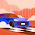4.5.Thank you for the valuable information,

6.Thank you sir Hamada.. its simple and easy to follow d contents.. thank you one again sir.. have a good day :)

7.8.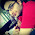Nice Explanation

9.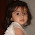Is it rectang?e hyperbola shape or not?

10.Is it rectang?e hyperbola shape or not?

11.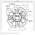Sir! I need a detail explanation on the topic: Electrical Drives